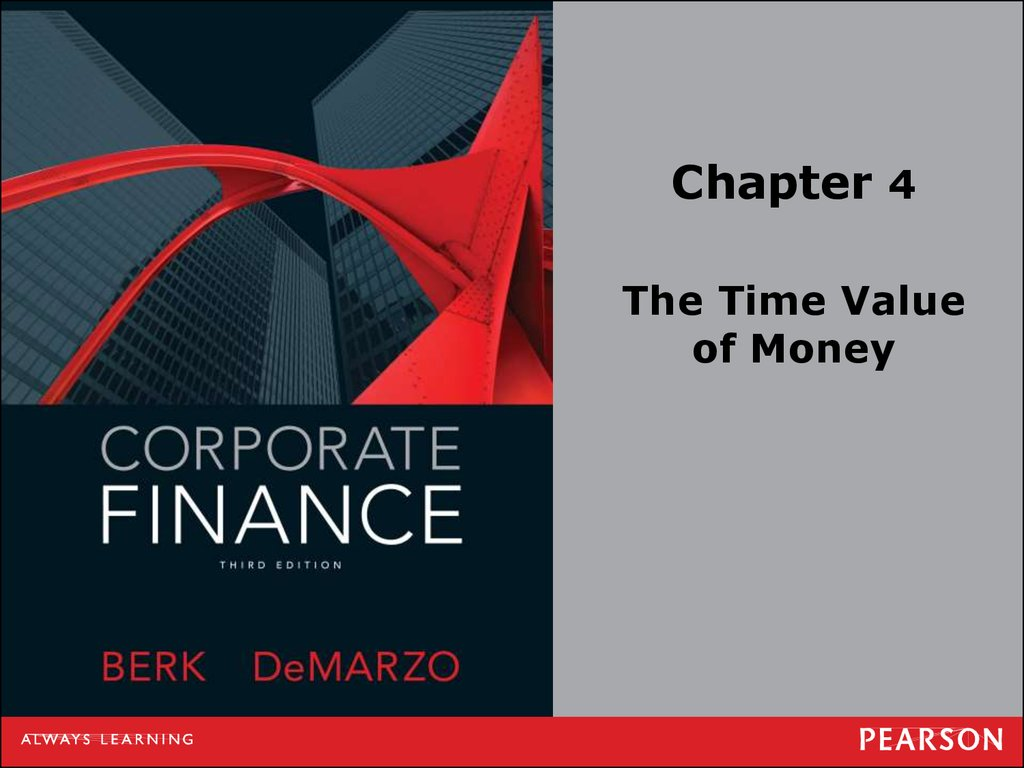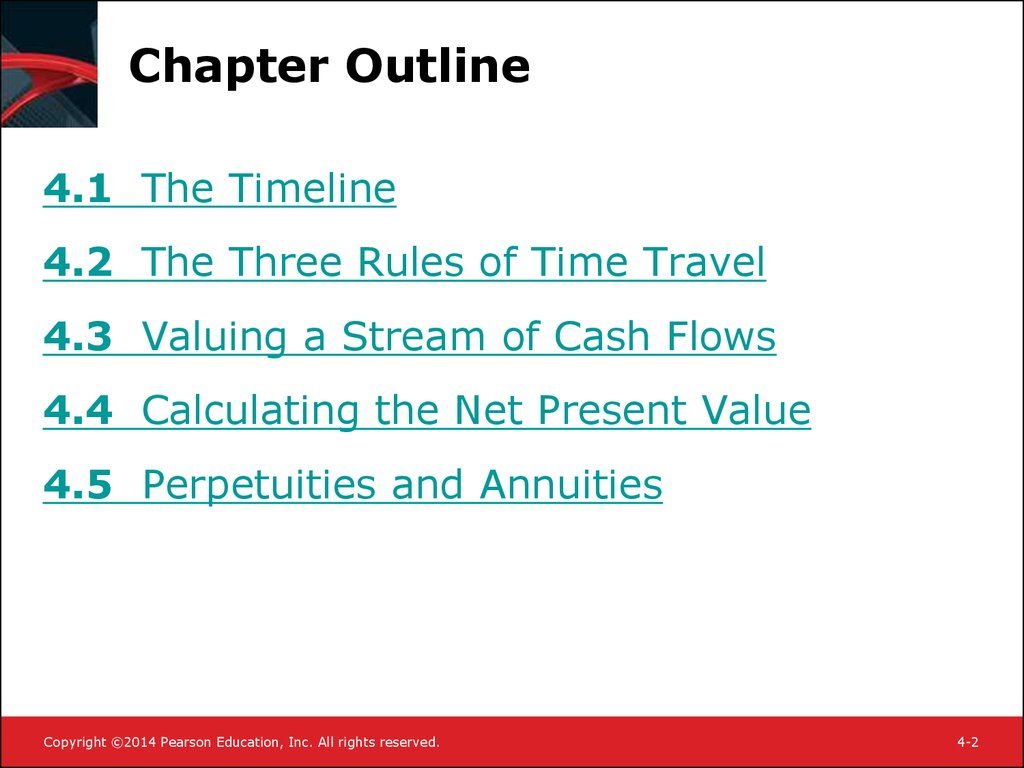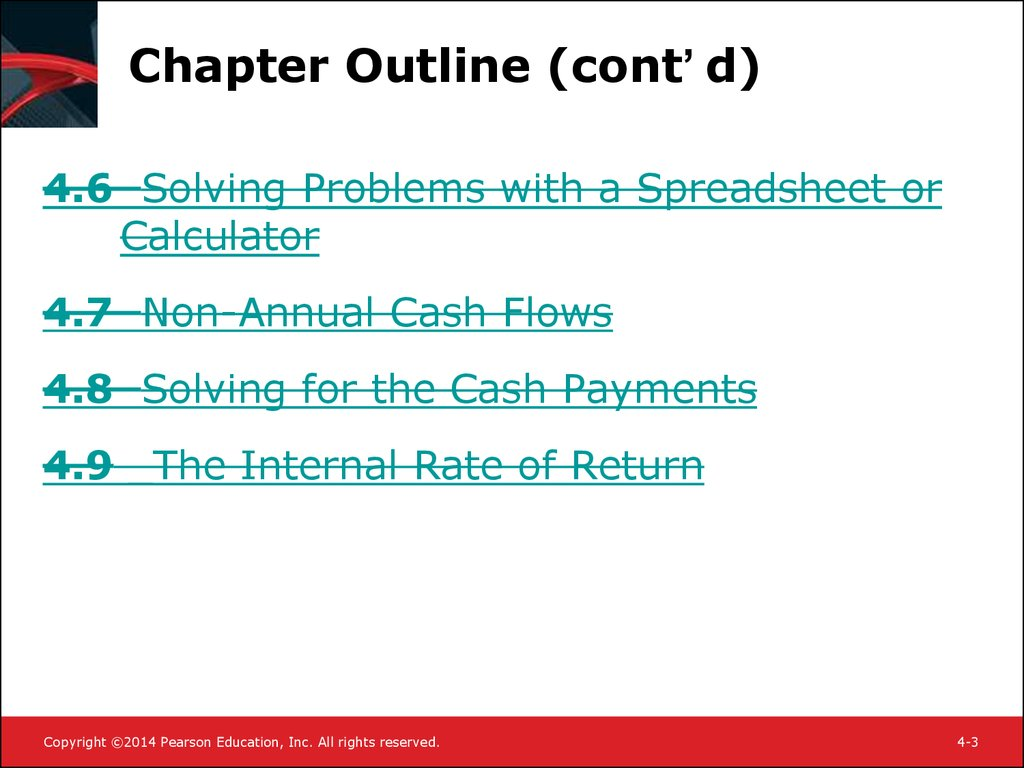# Chapter 4. The Time Value of Money

Chapter 4
The Time Value
of Money

## 2. Chapter Outline

4.1 The Timeline
4.2 The Three Rules of Time Travel
4.3 Valuing a Stream of Cash Flows
4.4 Calculating the Net Present Value
4.5 Perpetuities and Annuities
4-2

## 3. Chapter Outline (cont’d)

Chapter Outline (cont’ d)
4.6 Solving Problems with a Spreadsheet or
Calculator
4.7 Non-Annual Cash Flows
4.8 Solving for the Cash Payments
4.9 _The Internal Rate of Return
4-3

## 4. 4.1 The Timeline

• A timeline is a linear representation of the
timing of potential cash flows.
• Drawing a timeline of the cash flows will
4-4

## 5. 4.1 The Timeline (cont’d)

4.1 The Timeline (cont’ d)
• Assume that you made a loan to a friend.
You will be repaid in two payments, one at
the end of each year over the next two
years.
4-5

## 6. 4.1 The Timeline (cont’d)

4.1 The Timeline (cont’ d)
• Differentiate between two types of cash
flows
– Inflows are positive cash flows.
– Outflows are negative cash flows, which are
indicated with a – (minus) sign.
4-6

## 7. 4.1 The Timeline (cont’d)

• Assume that you are lending \$10,000 today and that the loan
will be repaid in two annual \$6,000 payments.
• The first cash flow at date 0 (today) is represented as a
negative sum because it is an outflow.
• Timelines can represent cash flows that take place at the end
of any time period – a month, a week, a day, etc.
4-7

## 8. 4.2 Three Rules of Time Travel

• Financial decisions often require combining
cash flows or comparing values. Three rules
govern these processes.
Table 4.1 The Three Rules of Time Travel
4-8

## 9. The 1st Rule of Time Travel

• A dollar today and a dollar in one year are
not equivalent.
• It is only possible to compare or combine
values at the same point in time.
– Which would you prefer: A gift of \$1,000 today
or \$1,210 at a later date?
– To answer this, you will have to compare the
alternatives to decide which is worth more. One
factor to consider: How long is “later?”
4-9

## 10. The 2nd Rule of Time Travel

• To move a cash flow forward in time, you
must compound it.
– Suppose you have a choice between receiving
\$1,000 today or \$1,210 in two years. You
believe you can earn 10% on the \$1,000 today,
but want to know what the \$1,000 will be worth
in two years. The time line looks like this:
4-10

## 11. The 2nd Rule of Time Travel (cont’d)

- Original capital: \$1,000
• Future Value of a Cash Flow
- Interest on original capital:
(1,000 x 10%) x 2 = \$200
- Interest on interest: 100 x
10% = \$10
- Total: 1,000+200+10 = 1,210
4-11

4-12

## 13. The 3rd Rule of Time Travel

• To move a cash flow backward in time, we
must discount it.
• Present Value of a Cash Flow
C
PV = C ¸ (1 + r ) =
n
(1 + r )
n
0
1
2
C
PV =
(1+ r )n

n-1
n
C
4-13

## 14. 4.3 Valuing a Stream of Cash Flows

• Based on the first rule of time travel we can
derive a general formula for valuing a
stream of cash flows: if we want to find the
present value of a stream of cash flows, we
simply add up the present values of each.
4-14

## 15. 4.3 Valuing a Stream of Cash Flows (cont’d)

• Present Value of a Cash Flow Stream
PV =
N
å PV(C )
n
n=0
=
N
å
n=0
Cn
(1 + r)n
4-15

## 16. 4.4 Calculating the Net Present Value

• Calculating the NPV of future cash flows
allows us to evaluate an investment
decision.
• Net Present Value compares the present
value of cash inflows (benefits) to the
present value of cash outflows (costs).
4-16

4-17

> 0 Accept!
4-18

## 19. 4.5 Perpetuities and Annuities

• Perpetuities
– When a constant cash flow will occur at regular
intervals forever it is called a perpetuity.
PV = ?
4-19

## 20. 4.5 Perpetuities and Annuities (cont’d)

• The value of a perpetuity is simply the cash
flow divided by the interest rate.
• Present Value of a Perpetuity
C
PV(C in perpetuity) =
r
PV = C/r
4-20

## 21. 4.5 Perpetuities and Annuities (cont’d)

4.5 Perpetuities and Annuities
(cont’ d)
• Annuities
– When a constant cash flow will occur at regular
intervals for a finite number of N periods, it is
called an annuity.
– Present Value of an Annuity
N
C
C
C
C
C
PV =
+
+
+... +

2
3
N
n
(1+ r) (1+ r ) (1+ r)
(1+ r )
(1+
r
)
n=1
4-21

## 22. Present Value of an Annuity

• For the general formula, substitute P for the
principal value and:
PV(annuity of Cfor N periods)
= P - PV(Pin period N)
é
ù
æ
ö
C
1
P
1
ú
= ê1= P= P ç1N
N
N ÷
r êë (1+ r ) úû
(1+ r)
è (1+ r) ø
4-22

## 23. Growing Cash Flows

• Growing Perpetuity
– Assume you expect the amount of your
perpetual payment to increase at a constant
rate, g.
• Present Value of a Growing Perpetuity
C
PV (growing perpetuity) =
r - g